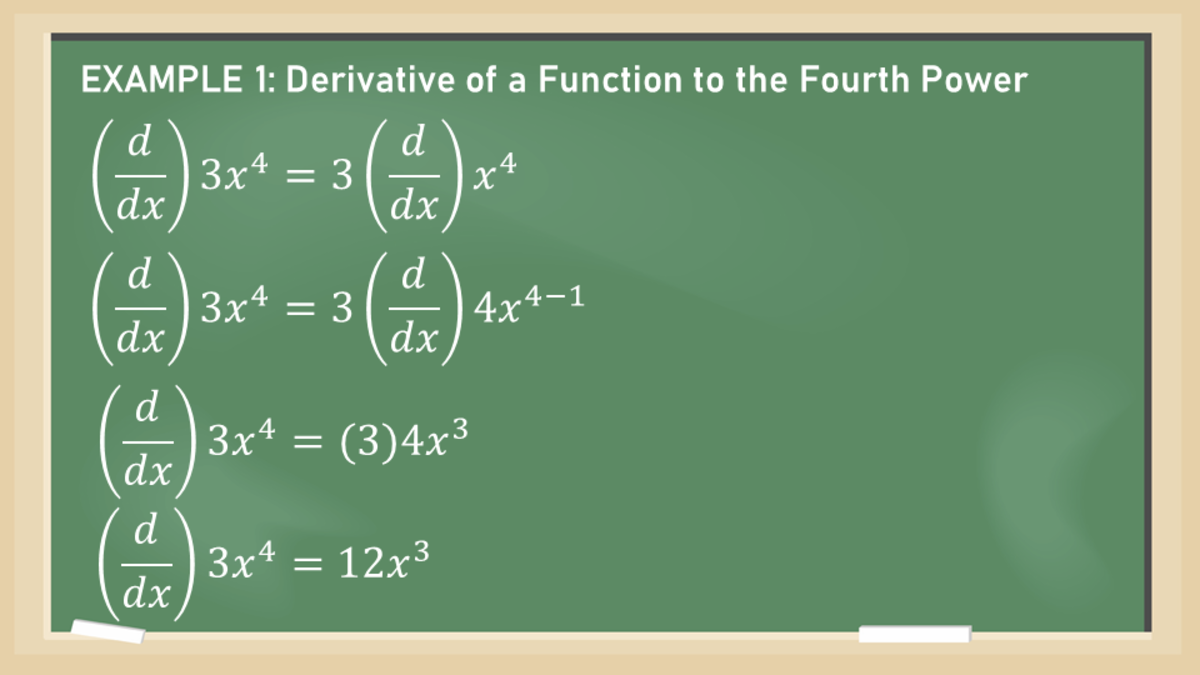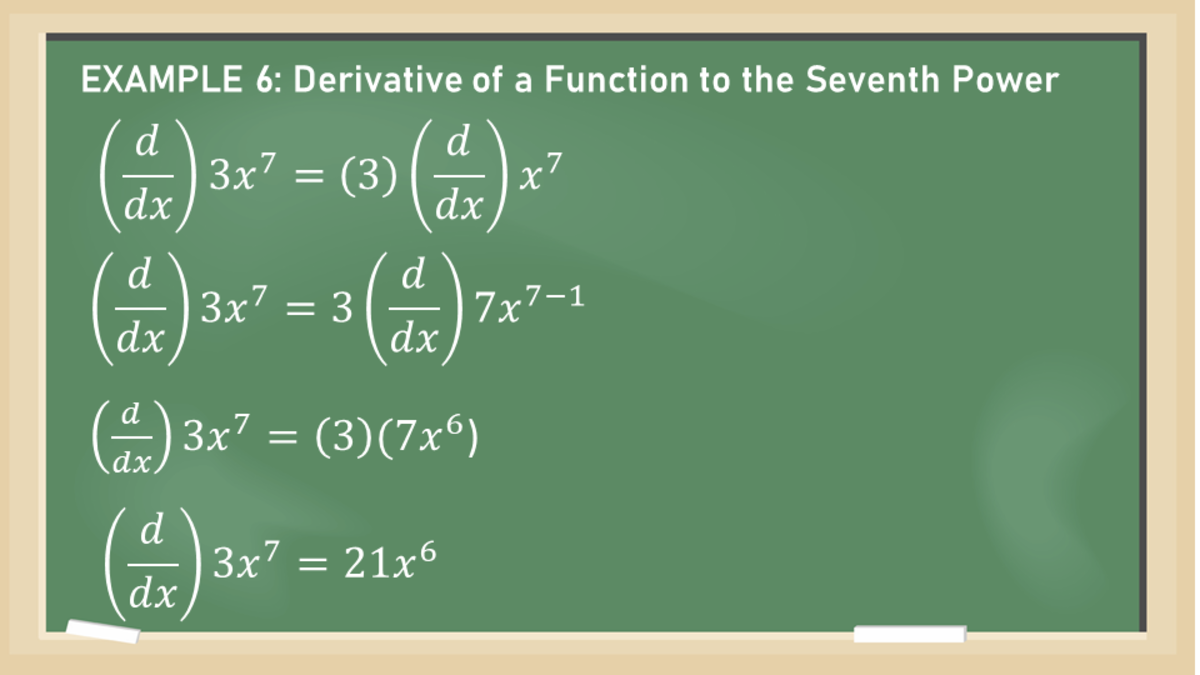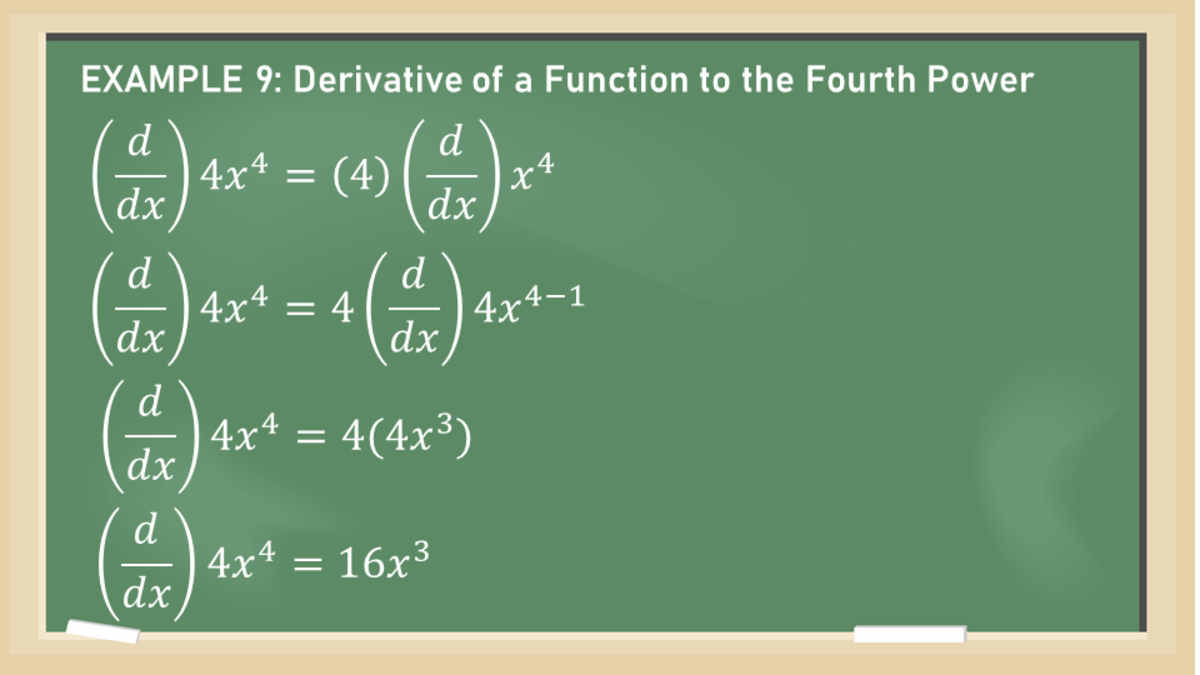# Constant Multiple Rule for Derivatives (With Proof and Examples)

• Author:
• Updated date:

Ray is a licensed engineer in the Philippines. He loves to write about mathematics and civil engineering.

## What Is the Constant Multiple Rule?

The derivative of constant times a function is the constant times the derivative of the function. If c is a constant and f is a differentiable function, then,

(d/dx) [c f(x)] = c (d/dx) [f(x)]

### Constant Multiple Rule Proof

When new functions are formed from old functions by multiplication by a constant or any other operations, their derivatives can be calculated using derivatives of the old functions. In particular, the Constant Multiple Rule states that the derivative of a constant multiplied by a function is the constant multiplied by the function's derivative.

Here is ample proof of Constant Multiple Rule using limits. Let g(x) = c f(x)

g’(x) = limh→o [g(x+h)-g(x)] / h

g’(x) = limh→o [cf(x+h)-cf(x)] / h

g’(x) = limh→o c [(f(x+h)-f(x)) / h]

g’(x) = c limh→o [(f(x+h)-f(x)) / h]

g’(x) = cf’(x)

Below is the geometric interpretation of the Constant Multiple Rule. Multiplying by c=2 stretches the graph vertically by a factor of 2. All the rises have been doubled, but the runs stay the same. So the slopes are doubled, too.

## Example 1: Derivative of a Function to the Fourth Power

Find the derivative of the function (d/dx) 3x4 using the Constant Multiple Rule.

Solution

Apply the Constant Multiple Rule by taking the derivative of the power function first and then multiply with the coefficient 3.

(d/dx) 3x4 = 3 (d/dx) x4

Scroll to Continue

Apply the Power Rule in differentiating the power function.

(d/dx) 3x4 = 3 (d/dx) 4x4-1

(d/dx) 3x4 = 3 (d/dx) 4x3

Simplify the algebraic expression.

(d/dx) 3x4 = 3 (d/dx) 4x3

(d/dx) 3x4 = 3(4x3)

(d/dx) 3x4 = 12x3

The derivative of the function (d/dx) 3x4 is 12x3.Constant Multiple Rule: Derivative of a Function to the Fourth Power

## Example 2: Derivative of a Negative Function

What is the derivative of the function (d/dx) -x?

Solution

Apply the Constant Multiple Rule by taking the derivative of the power function first and then multiply with the coefficient -1.

(d/dx) -x = (d/dx) [(-1) x]

Apply the Power Rule in differentiating the power function.

(d/dx) -x = (-1) (d/dx) x

Recall that the derivative of x is 1. Simplify further the algebraic expression.

(d/dx) -x = -1(1)

(d/dx) -x = -1

The derivative of the function -x is -1.

## Example 3: Derivative of a Fraction

What is the derivative of the function y= (1/2) x?

Solution

Apply the Constant Multiple Rule by taking the derivative of the power function first and then multiply with the coefficient \frac {1} {2}. Apply the Power Rule in differentiating the power function.

(d/dx) (1/2)x = (1/2) (d/dx) x

Recall that the derivative of x is 1. Simplify further the algebraic expression.

(d/dx) (1/2) x = (1/2) (1)

(d/dx) (1/2) x = ½

The derivative of the function y = (1/2) x is ½.

## Example 4: Derivative of a Function of X

Find the derivative of the constant multiple function f(x)=6x.

Solution

Apply the Constant Multiple Rule by taking the derivative of the power function first and then multiply with the coefficient 6. Apply the Power Rule in differentiating the power function.

(d/dx) 6x = 6 (d/dx) x

Recall that the derivative of x is 1. Simplify further the algebraic expression.

(d/dx) 6x = 6(1)

(d/dx) 6x = 6

The derivative of the function f(x)=6x is 6.

## Example 5: Derivative of a Complex Cube Root Function

What is the derivative of the constant multiple function y = (3√8) x3?

Solution

Apply the Constant Multiple Rule by taking the derivative of the power function first and then multiply with the coefficient 3√8. Apply the Power Rule in differentiating the power function.

(d/dx) (3√8) x3 = (3√8) (d/dx) x3

Recall the Power Rule and solve for the derivative of the power function x3.

(d/dx) (3√8) x3 = 3√8 (3x2)

(d/dx) (3√8) x3 = 2(3x2)

(d/dx) (3√8) x3 = 6x2

The derivative of the function y = (3√8) x3 is 6x2.

## Example 6: Derivative of a Function to the Seventh Power

Find the derivative of the function y = 3x7 using the Constant Multiple Rule.

Solution

First, separate the constant value of 3 from the whole function. Then, take the derivative of the power function x7 using the Power Rule.

(d/dx) 3x7 = 3 (d/dx) x7

Apply the Power Rule then multiply the result to the constant 3, as stated from the Constant Multiple Rule.

(d/dx) 3x7 = 3 (d/dx) x7 = 3(7x7-1)

(d/dx) 3x7 = 3(7x6)

(d/dx) 3x7 = 21x6

The derivative of the function y = 3x7 is 21x6.Constant Multiple Rule: Derivative of a Function to the Seventh Power

## Example 7: Derivative of a Negative Function

What is the derivative of the constant multiple function (d/dx) (-5x)?

Solution

From the given function, segregate the negative value constant from the x variable.

(d/dx) -5x= -5 (d/dx) x

Apply the Power Rule, then multiply the outcome with a negative five.

(d/dx) -5x= -5 (d/dx) x

(d/dx) -5x= -5 (d/dx) x1-1

(d/dx) -5x= -5 (d/dx) x0

(d/dx) -5x= -5 (d/dx) (1)

(d/dx) -5x= -5

The derivative of the function y = -5x is -5.

## Example 8: Derivative of a Pi Function

Find the derivative of the function y = 3πt.

Solution

The given function is a radian function of variable t. Recall that pi is a constant value of 3.14. It means that the part with 3π will be the constant of the pi function. Separate the constant value 3π from the variable t and differentiate t alone.

(d/dt) 3πt= 3π (d/dt) t

Apply the Power Rule and the Constant Multiple Rule to the equation.

(d/dt) 3πt= 3π (d/dt) t1-1

(d/dt) 3πt= 3π (d/dt) t0

(d/dt) 3πt= 3π

The derivative of the function y = 3πt is 3π.

## Example 9: Derivative of a Function to the Fourth Power

Solve for the derivative of the function f(x) = 4x4.

Solution

In getting the derivative of the function 4x4, the Constant Multiple Rule applies. Like the usual method, separate the constant four from x4 and then solve the power function's derivative.

(d/dx) 4x4= 4 (d/dx) x4

Apply the Power Rule and the Constant Multiple Rule to the equation.

(d/dx) 4x4= 4 (d/dx) x4

(d/dx) 4x4= 4 (d/dx) 4x4-1

(d/dx) 4x4= 4 (d/dx) 4x3

(d/dx) 4x4= 16x3

The derivative of the function 4x4 is 16x3.Constant Multiple Rule: Derivative of a Function to the Fourth Power

## Example 10: Derivative of a Sum of Power Functions

Find the derivative of the function f(x) = 6x3 + 9x2 + 2x + 8.

Solution

The given equation is a run of power functions. To solve, differentiate the terms individually. Start with the 6x3 and apply the Constant Multiple Rule.

(d/dx) 6x3= 6 (d/dx) x3

(d/dx) 6x3= 6 (3x3-1)

(d/dx) 6x3= 6 (3x2)

(d/dx) 6x3= 18x2

(d/dx) 9x2= 9 (d/dx) x2

(d/dx) 9x2= 9 (2x2-1)

(d/dx) 9x2= 9 (2x1)

(d/dx) 9x2= 18x

(d/dx) 2x= 2 (d/dx) x

(d/dx) 2x = 2 (x1-1)

(d/dx) 2x = 2 (x0)

(d/dx) 2x = 2 (1)

(d/dx) 2x = 2